• 2
•
• 2
•
• 2
•
•
•
6
Shares

# MAGNETIC FIELD DUE TO SOLENOID DERIVATION CLASS-12

## OVERVIEW

A solenoid is a helical coil that can be obtained by tightly bounding the copper wire on the cylindrical portion of the insulating material. When electric current flows through the solenoid, it creates a magnetic field.

The direction of the magnetic field can be given by using the right-hand thumb rule. When you curl your right hand’s finger holding the wire such that your thumb points the direction of electric current then your curl fingers give the direction of the magnetic field.

The solenoid is used in the experimental work where a controlled magnetic field is required. Suggested reading: Solenoid and their applications.

## MAGNETIC FIELD OF A SOLENOID

We know that when we supply electric current to the solenoid, it creates the magnetic field and we also know that its direction can be given by the right-hand thumb rule.

The orientation of the magnetic field produced by the solenoid is the same as the orientation of the magnetic field produced by the bar magnet. Due to this resemblance of magnetic field lines, a solenoid is also taken as a bar magnet. Because it is like a bar magnet so it has both properties of attraction and repulsion.

Magnetic field lines normally exit and enter through the cross-section of solenoid poles and it is uniform and parallel within the solenoid. The strength of the magnetic field is maximum at the center of the solenoid.

In this article, we are going to derive an expression for the magnetic field due to a solenoid. Here, we will derive the expression for the magnetic field in two ways first using Biot-Savart Law and second using Ampere’s circuital law. So let’s get started…

## DERIVATION OF MAGNETIC FIELD OF A SOLENOID USING BIOT-SAVART LAWFig. 2, labeled diagram to find the magnetic field at the point P, source: phys.libretexts.org

Let’s consider a solenoid consisting of N turns of wire tightly wound over a length L. A current I is flowing through the solenoid. A point P lies on the solenoid’s central axis where we want to find the value of the magnetic field. The number of turns per unit length of the solenoid is N/L, therefore, the number of turns in an infinitesimal length dy is (N/L)dy turns. The current in this infinitesimal length (dy) of the wire is given as-

(1)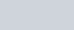Now, let’s calculate the magnetic field at the point P that lies on the central axis of the solenoid. To do so, first cut the solenoid into several small slices that are of thickness (dy) and treat each slice as a current carrying circular loop. If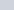is the current flowing through each slice. Then the magnetic field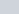due to currentin dy is given using Biot-Savart Law is as-In the above equation, we have replacedwith its expression which is given in equ(1). The resultant field at P can be obtained by integratingalong the entire length of the solenoid. It’s easiest to evaluate this integral by changing the independent variable from y to θ. From the inspection of the above diagram, we find that-

(2)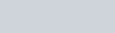Taking differential on the both side of the equ(2), we get-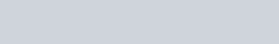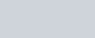When we substitute this value in the expression for, we get-This is the magnetic field along the central axis of a finite solenoid. But for a special solenoid whose length is infinite, means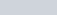. From the practical point of view a infinite solenoid is one whose length is much larger than its radius (L>>>>R), then in this case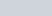and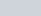, putting these values of θ in above the equation, then the magnitude of magnetic field due to an infinitely long solenoid is given as-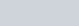where n is numbers of turns per unit length.

## DERIVATION OF MAGNETIC FIELD OF A SOLENOID USING AMPERE’S CIRCUITAL LAW

In this section, we will derive the expression of the magnetic field using Ampere’s circuital law. To derive the magnetic field using Ampere’s circuital law, let’s take a rectangular closed path called amperian loop, A-B-C-D-A such that its half part lies within the solenoid and half remains outside the solenoid. See figure below:

Along the segment AB,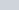is uniform and parallel to the path. Along with segments BC and DA,is perpendicular to part of the path and vanishes over the rest of it. Therefore, segments BC and DA do not contribute to the line integral in Ampère’s circuital law. Along segment CD,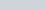because the magnetic field is zero outside the solenoid.

If you analyze an analysis of an Ampère’s law loop outside of the solenoid, you find that the current flows in opposite directions on different segments of wire. Therefore, there is no enclosed current and so no magnetic field according to Ampère’s circuital law. Thus, there is no contribution to the line integral from segment CD.So this is the required derivation of the magnetic field on the central axis of a solenoid. Here, the location of segment AB is arbitrary, so we have found that this equation gives the magnetic field everywhere inside the infinite solenoid. Stay tuned with Laws Of Nature for more useful and interesting content.